## Print probabilities or biases (polarizations) of individual qubits

The commands below internally use the same routine as that for calculating reduced density operators (See Sec. 10). Let the reduced density operator of the i-th qubit be. Then the probability of the i-th qubit being 0 is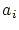, and the superficial bias of it is defined as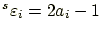. The values ofand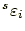may be changed even by single-qubit rotation gates. On the other hand, there is a definition of polarization that is independent of a macroscopic spin direction: Let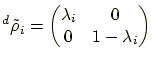be the matrix obtained from a unitary diagonalization of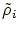(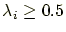). Then the intrinsic bias of the qubit is defined as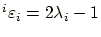.

1. Every probability of each specified qubit being measured as 0 is printed by the command:
bprobs (bit labels);
2. All the probabilities of individual qubits being measured as 0 are printed by the command:
bprobs;
3. The intrinsic biases of specified individual qubits are printed by the command:
ibiases (bit labels);
4. All the intrinsic biases of individual qubits are printed by the command:
ibiases;
5. The superficial biases of specified individual qubits are printed by the command:
sbiases (bit labels);
6. All the superficial biases of individual qubits are printed by the command:
sbiases;
Example:
	 > init-mixed 0.6 0.7 0.8;
> bprobs(1-3);
1:0.6 2:0.7 3:0.8
> sbiases;
1:0.2 2:0.4 3:0.6
> H(2);
> sbiases;
1:0.2 2:0 3:0.6
> ibiases;
1:0.2 2:0.4 3:0.6

root
2004-11-07